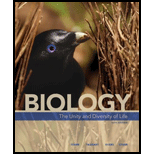Chapter 44, Problem 9SQ### Biology: The Unity and Diversity o...

15th Edition
Cecie Starr + 3 others
ISBN: 9781337408332

#### Solutions

Chapter
Section### Biology: The Unity and Diversity o...

15th Edition
Cecie Starr + 3 others
ISBN: 9781337408332
Textbook Problem
1 views

# The human population is now about 7 billion. It reached 6 billion in _____. a. 2007 b. 1999 c. 1802 d. 1350

Summary Introduction

Concept introduction: Population is the total number of organisms present in a specified area and they are capable of interbreeding. Demography is a study of characteristics of the human population about its size, density, and distribution. It is a statistical interrelationship between the population and economic development.

Explanation

Reasons for correct statements:

Demographic studies reveal the statistical composition of a particular human population. The human population is estimated as 7.62 billion as of May 2018.

Option b. is given as, “1999.” Demographic studies estimated that the global population of human was 6 billion. Hence the correct answer is option b.

Reasons for incorrect statements:

Option a. is given as, “2007”...

### Still sussing out bartleby?

Check out a sample textbook solution.

See a sample solution

#### The Solution to Your Study Problems

Bartleby provides explanations to thousands of textbook problems written by our experts, many with advanced degrees!

Get Started